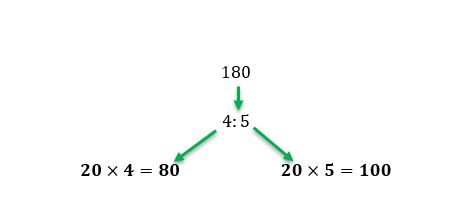# How to divide ratios

Want to find out how to divide ratios? We've provided a step-by-step guide of how to divide ratios to teach you everything you need to know.## Step 1: Find the sum of the ratios values

To divide an amount given a ratio, find the sum of the ratio’s values. This means that to divide 132 to 5:6, we first add 5 and 6.

## Step 2: Divide by the sum of the ratio

Divide the given amount by the sum of the ratio. Hence, we divide 132 by 11.

## Step 3: Multiply with the quotient

Divide the amount to the given ratios by multiplying the ratio’s values with the quotient from Step 2. Hence, when dividing 132 into the ratio of 5:6, we have 60 and 72.

## Examples of dividing ratios

Q1) Harry has 180 new Pokémon cards and plans to share this with his sister. The cards will be divided into the ratio of 4:5, where Harry gets more cards. How many cards will each have?

We want to divide 180 into the ratio of 4:5. So, we add their ratios first and have 4+5=9

Once we have the sum of the ratios, we divide 180 by 9. Once we have the quotient, multiply 20 by the ratio.This means that Harry is going to have 100 cards and his sister gets 80 cards.

Q2) Jack plans to give his three children a total of \$1440. The amount will be divided in the ratio of 1:2:3, with the youngest receiving the least amount. How much would each Jack’s child receive?

To divide 1440 into the ratio of 1:2:3, we add the three values of the ratio and have

1+2+3=6

Now, divide the amount by the sum of the ratios.

1440÷6=240

Multiply the quotient back to each of values in the ratio.

1×240=240 2×240=480 3×240=720

This means that the youngest child receives \$240, the middle child receives \$480, and youngest child receives \$720.

#### Join today

The fastest way to practice

Unlock our complete testing platform and improve faster that ever.